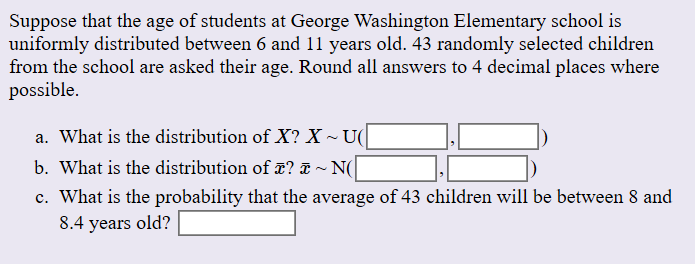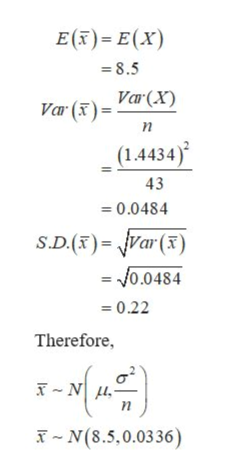# Suppose that the age of students at George Washington Elementary school isuniformly distributed between 6 and 11 years old. 43 randomly selected childrenfrom the school are asked their age. Round all answers to 4 decimal places wherepossiblea. What is the distribution of X? XU(b. What is the distribution of ? & ~ N(c. What is the probability that the average of 43 children will be between 8 and8.4 years old?

Question
197 viewshelp_outlineImage TranscriptioncloseSuppose that the age of students at George Washington Elementary school is uniformly distributed between 6 and 11 years old. 43 randomly selected children from the school are asked their age. Round all answers to 4 decimal places where possible a. What is the distribution of X? X U( b. What is the distribution of ? & ~ N( c. What is the probability that the average of 43 children will be between 8 and 8.4 years old? fullscreen
check_circle

Step 1
1. It is provided that the age of students is uniformly distributed between 6 and 11 years old. The randomly selected sample, n is 43.

The uniform distribution of X can be obtained as:

Step 2

The mean and standard deviation of X for the uniform distribution is,

Step 3

b. The Central Limit Theorem states that if the sample size is greater than or equal to 30, the sampling distributions of the mean tend to be normal distribution, irre...help_outlineImage TranscriptioncloseE() E(x) 8.5 Var ()= Var(X) п (1.4434) 43 0.0484 S.D.() var() = 0.0484 0.22 Therefore, п TN(8.5,0.0336) fullscreen

### Want to see the full answer?

See Solution

#### Want to see this answer and more?

Solutions are written by subject experts who are available 24/7. Questions are typically answered within 1 hour.*

See Solution
*Response times may vary by subject and question.
Tagged in